# CBSE Class 3 Maths Worksheet- Give And Take Notes | Study Mathematics for Class 3: NCERT - Class 3

## Class 3: CBSE Class 3 Maths Worksheet- Give And Take Notes | Study Mathematics for Class 3: NCERT - Class 3

The document CBSE Class 3 Maths Worksheet- Give And Take Notes | Study Mathematics for Class 3: NCERT - Class 3 is a part of the Class 3 Course Mathematics for Class 3: NCERT.
All you need of Class 3 at this link: Class 3

Q.1. Subtraction
(i) 10  -  7  =  _____
Ans.
3

(ii) 28  -   6  =  _____
Ans.
22

(iii) 19  -  9  =  _____
Ans.
10

(iv) 9  -  4  =  _____
Ans.
5

(v) 18  -  5  =  _____
Ans.
13

(vi) 15  -  3  =  _____
Ans.
12

(vii)  20  -  5  =  _____
Ans.
15

(viii)  33  -  3  =  _____
Ans.
30

(ix)  _____  -  7  =  11
Ans.
18

(x)  _____  -  5  = 6
Ans.
11

(xi) _____  -  4  =  8
Ans.
12

(xii) _____  -  6  =  9
Ans.
15

(xiii) _____  -  4  =  14
Ans.
18

(xiv) _____  -  1  =  16
Ans. 17

(xv) 29  -  _____  =  22
Ans.
7

(xvi) 40  -  _____  =  32
Ans.
8

(xvii)  15  -  _____  =  11
Ans.
4

(xviii)  9  -  _____  =  0
Ans.
9

(xix) 28  -  _____  =  18
Ans.
10

(xx)  37  -  _____  =  25
Ans.
12

Q.2. Subtract to find the differences.
(a)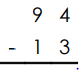Ans.
8   1

(b)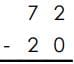Ans.
5   2

(c)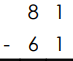Ans.
2   0

(d)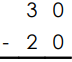Ans.
1   0

(e)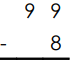Ans.
9   1

(f)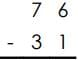Ans.
4   5

(g)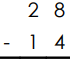Ans.
1   4

(h)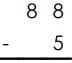Ans.
8   3

(i)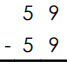Ans.
0

(j)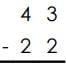Ans.
2  1

(k) Amy has 36 markers. 12 do not work.How many markers do work?
Ans.
24 markers

(l) There are 25 kids in Mr. Ying's class. 13 are girls. How many are boys?
Ans.
12 boys

Q.3.
(i) 31  +  7  =  _____
Ans.
38

(ii) 28  +  3  =  _____
Ans.
31

(iii) 64  +  9  =  _____
Ans.
73

(iv) 56  +  4  =  _____
Ans.
60

(v) 42  +  9  =  _____
Ans.
51

(vi) 15  +  8  =  _____
Ans.
23

(vii)  23  +  5  =  _____
Ans.
28

(viii)  71  +  3  =  _____
Ans.
74

(ix)  40  +  7  =  _____
Ans.
47

(x)  83  +  5  =  _____
Ans.
88

(xi) 96  +  4  =  _____
Ans.
100

(xii) 77  +  6  =  _____
Ans.
83

(xiii) 25  +  9  =  _____
Ans.
34

(xiv) 57  +  3  =  _____
Ans.
60

(xv) 79  +  1  =  _____
Ans.
80

(xvi) 19  +  8  =  _____
Ans.
27

(xvii)  67  +  7  =  _____
Ans.
74

(xviii)  49  +  9  =  _____
Ans.
58

(xix) 90  +  8  =  _____
Ans.
98

(xx)  35  +  2  =  _____
Ans.
37

The document CBSE Class 3 Maths Worksheet- Give And Take Notes | Study Mathematics for Class 3: NCERT - Class 3 is a part of the Class 3 Course Mathematics for Class 3: NCERT.
All you need of Class 3 at this link: Class 3Use Code STAYHOME200 and get INR 200 additional OFF

## Mathematics for Class 3: NCERT

57 videos|70 docs|44 tests

Track your progress, build streaks, highlight & save important lessons and more!

,

,

,

,

,

,

,

,

,

,

,

,

,

,

,

,

,

,

,

,

,

;